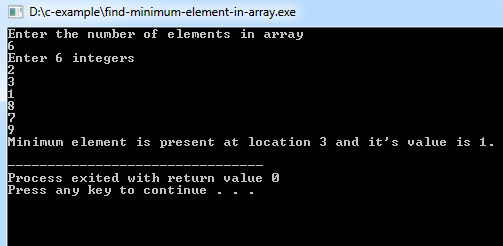# C Program to Find Minimum Element in Array

C program to find smallest element present in an array. It also prints the location or index at which minimum element occurs in array.

Program:
``````#include <stdio.h>

int main()
{
int array, minimum, size, c, location = 1;

printf("Enter the number of elements in array\n");
scanf("%d",&size);

printf("Enter %d integers\n", size);

for ( c = 0 ; c < size ; c++ )
scanf("%d", &array[c]);

minimum = array;

for ( c = 1 ; c < size ; c++ )
{
if ( array[c] < minimum )
{
minimum = array[c];
location = c+1;
}
}

printf("Minimum element is present at location %d and it's value is %d.\n", location, minimum);
return 0;
}``````
Program Output:## Same C program using pointers

Program:
``````#include <stdio.h>

int main()
{
int array, *minimum, size, c, location = 1;

printf("Enter the number of elements in array\n");
scanf("%d",&size);

printf("Enter %d integers\n", size);

for ( c = 0 ; c < size ; c++ )
scanf("%d", &array[c]);

minimum = array;
*minimum = *array;

for ( c = 1 ; c < size ; c++ )
{
if ( *(array+c) < *minimum )
{
*minimum = *(array+c);
location = c+1;
}
}

printf("Minimum element found at location %d and it's value is %d.\n", location, *minimum);
return 0;

}``````

The algorithm first assumes the first element as a minimum, and then compare it with other elements if an element it is smaller than the new minimum and the entire array is scanned until the process is repeated.